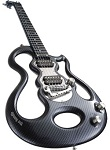Welcome to CustomsForge Forums
Register now to gain access to all of our features. Once registered and logged in, you will be able to create topics, post replies to existing threads, give reputation to your fellow members, get your own private messenger, post status updates, manage your profile and so much more. This message will be removed once you have signed in.
Login to Account Create an Account

### Learn & Play Rocksmith!

Want to play the songs you love? Registration and the use of this website is 100% free.
The only thing you need is a legal copy of Rocksmith 2014 w/ a cable. Click here to buy it.

Having trouble logging in or registering? Please click "Support" below.

### Chlipouni# Chlipouni

Member Since 17 Feb 2014
OFFLINE Last Active Yesterday, 08:54 AM### In Topic: Latest EOF releases (2-20-2019)

28 September 2018 - 08:22 AM

@raynebc,

I don't remember if "Import/Lyrics" works with accented characters.

If my input lyrics file contains "é", it is not imported correctly in EOF. If I directly type it in EOF, it is correctly displayed.

Do I need to use a special encoding setting for my input file ?

Thanks

### In Topic: Lyric's bar improvements

24 September 2018 - 01:55 AM

It is mainly about the highlighting of the selected graphical bar in the piano roll area.

Thanks

### In Topic: Lyric's bar improvements

23 September 2018 - 05:14 PM

I have no specific preference for the selected bar colour.

Just something that highlights them more ...

### In Topic: Latest EOF releases (2-20-2019)

18 June 2018 - 12:45 AM

@raynebc,

The logic seems fine for me.

I didn't know what action to use to remove the warning message.

Thanks

### In Topic: Latest EOF releases (2-20-2019)

16 June 2018 - 02:49 AM

@raynebc,

I tried the beta release with the following example :

EOF - Chords - HandShapes 2

The XML code is :

```    <level difficulty="0">
<notes count="3">
<note time="6.443" string="1" fret="3" sustain="1.088" slideTo="7" />
<note time="6.443" string="2" fret="5" sustain="1.088" slideTo="9" />
<note time="6.443" string="3" fret="5" sustain="1.088" slideTo="9" />
</notes>
<chords count="2">
<chord time="5.352" chordId="0" linkNext="1" >
<chordNote time="5.352" string="1" fret="3" sustain="1.091" linkNext="1" leftHand="1" />
<chordNote time="5.352" string="2" fret="5" sustain="1.091" linkNext="1" leftHand="3" />
<chordNote time="5.352" string="3" fret="5" sustain="1.091" linkNext="1" leftHand="4" />
</chord>
<chord time="7.534" chordId="1" >
<chordNote time="7.534" string="1" fret="7" leftHand="1" />
<chordNote time="7.534" string="2" fret="9" leftHand="3" />
<chordNote time="7.534" string="3" fret="9" leftHand="4" />
</chord>
</chords>
<fretHandMutes count="0"/>
<anchors count="1">
<anchor time="5.352" fret="3" width="7.000"/>
</anchors>
<handShapes count="2">
<handShape chordId="0" startTime="5.352" endTime="7.531"/>
<handShape chordId="1" startTime="7.534" endTime="8.080"/>
</handShapes>
</level> ```

The first handShape tag includes correctly the second chord, so this problem is solved.

As you can see, I added a third chord that starts on the 7 fret. This new chord is not fully included in the current anchor tag, but EOF doesn't generate a new anchor tag for it.

Instead of that, EOF displays this warning : "This note is specified as using the index finger, but the first hand position is on a different fret....".

Is it normal ?

If yes, how can I solve this ?

If I remove the linkNext status on the first chord, the new XML code becomes :

```    <level difficulty="0">
<notes count="0"/>
<chords count="3">
<chord time="5.352" chordId="0" >
<chordNote time="5.352" string="1" fret="3" leftHand="1" />
<chordNote time="5.352" string="2" fret="5" leftHand="3" />
<chordNote time="5.352" string="3" fret="5" leftHand="4" />
</chord>
<chord time="6.443" chordId="0" >
<chordNote time="6.443" string="1" fret="3" sustain="1.088" slideTo="7" leftHand="1" />
<chordNote time="6.443" string="2" fret="5" sustain="1.088" slideTo="9" leftHand="3" />
<chordNote time="6.443" string="3" fret="5" sustain="1.088" slideTo="9" leftHand="4" />
</chord>
<chord time="7.534" chordId="1" >
<chordNote time="7.534" string="1" fret="7" leftHand="1" />
<chordNote time="7.534" string="2" fret="9" leftHand="3" />
<chordNote time="7.534" string="3" fret="9" leftHand="4" />
</chord>
</chords>
<fretHandMutes count="0"/>
<anchors count="1">
<anchor time="5.352" fret="3" width="7.000"/>
</anchors>
<handShapes count="3">
<handShape chordId="0" startTime="5.352" endTime="6.440"/>
<handShape chordId="0" startTime="6.443" endTime="7.531"/>
<handShape chordId="1" startTime="7.534" endTime="8.080"/>
</handShapes>
</level>
```

In this specific case, EOF creates a new handShape to manage the second chord, but it should be able to reuse the previous handShape (because they both have the same chordId).

Thank you